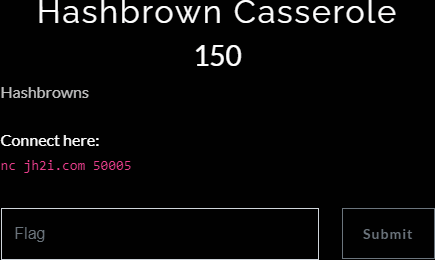# Hacktivitycon CTF: Hashbrown Casserole

So hacktivitycon CTF has ended. And my team, OpenToAll, took third place.

I ended up solving a scripting challenge by the name of `Hashbrown Casserole`. (I'm going to neatly evade the whole checksum vs hash distrinction for the sake of brevity.)

Here's what I was given:When I connected to the server this was the prompt:

``````Enter the data required for the first part of the md5sum to equal hex: 7d7519
``````

So they've given the task of finding the original data that lead to a hash. Luckily for us we only need to match the first 6 chars.

We're going to just generate random data...

``````def random_data():
start = 0
stop = 0xffffffffffffffffffffffff   # 96 bit length
data = random.randrange(start, stop)
return data # returns int``````

And compare it to the partial hash given until we find a match.

``````    partial_hash = '7d7519'
hash = ''

while(hash[:6] != partial_hash):
data = random_data()
hash = hashlib.md5(data.to_bytes(12, 'big')).hexdigest()``````

Though it took some time and computing power, we've got the data and send it to the server thru the socket:

``````Nope. :(
``````

Hmmf... Our data is int form `77971137074985976270646761813`. When we turn it to binary: `b'\xfb\xf06\x82d\xab\x8e\xa7\xb2eYU'` and send it the server finally responds:

``````Correct.
``````

Bad news is we're are given another prompt:

``````Enter the data required for the first part of the sha1sum to equal hex: 17f86b
``````

The fun never stops. Now they've given us a twist. They've changed the hashing algorithm to sha1!

So we changed our script accordinly, and pattern matched for not only the partial hash but also the algorithm type. We rewrote our function. And we added a loop and run the script. We achieved success after to success and I watch this run and wonder when the hell we'll be given the flag. Each hash takes some time and I'm left to wonder how long this all going to take. But our script fails:

``````Enter the data required for the first part of the sha1sum to equal hex: 93a41

Nope. :(
``````

Twist #2: Most times the partial hash given has a length of 6. But sometimes it's 5! But that's okay. We adjust our script and run it once more. I leave it alone and wondering if I'm missing something.

When I come back I see the thing has looped 50 times but finally we get a new response:

``````That casserole was DELICIOUS!!!! Here's your flag: flag{warm_casseroles_for_breakfast!!!}
``````

Turns out it takes 50 hashes to make a casserole. Who'da thunk it?

Here's the final script:

``````import hashlib
import random
import time
from pwn import *
import re

context(arch = 'i386', os = 'linux')
HOST = 'jh2i.com'
PORT = 50005

def random_data():
start = 0
stop = 0xffffffffffffffffffffffff   # 96 bit length
data = random.randrange(start, stop)
return data # returns int

def compare_checksum(source, hash_type, partial_hash):
hash_len = len(partial_hash)
hash_func = getattr(hashlib, hash_type) # sha1 or md5?

digest = hash_func(source.to_bytes(12, 'big')).hexdigest()
if digest[:hash_len] == partial_hash:
return digest
return False

def find_data_matching_checksum(hash_type, partial_hash):
res = False
while (res == False):
test_data = random_data();
res = compare_checksum(test_data, hash_type, partial_hash)
return test_data

def main():
re_prompt = r"([a-zA-z0-9]+)sum.*hex: ([a-f0-9]+)"

r = remote(HOST, PORT)
while True:
data = r.recvline()

data = data.decode('ascii').strip()
print('>', data)

if re.match(r"^Enter the data", data):
result = re.findall(re_prompt, data)
hash_type = result
partial_hash = result

print(hash_type, partial_hash, len(partial_hash))

start = time.time()

data = find_data_matching_checksum(hash_type, partial_hash)

end = time.time()
execution_time = end - start

answer = pack(data, 'all', 'big')